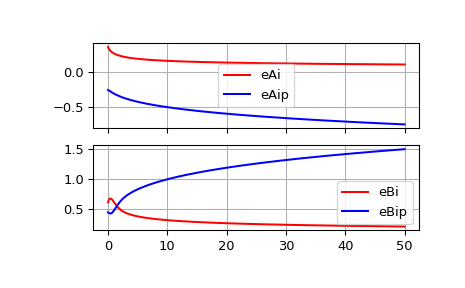# scipy.special.airye¶

scipy.special.airye(z) = <ufunc 'airye'>

Exponentially scaled Airy functions and their derivatives.

Scaling:

eAi  = Ai  * exp(2.0/3.0*z*sqrt(z))
eAip = Aip * exp(2.0/3.0*z*sqrt(z))
eBi  = Bi  * exp(-abs(2.0/3.0*(z*sqrt(z)).real))
eBip = Bip * exp(-abs(2.0/3.0*(z*sqrt(z)).real))

Parameters
zarray_like

Real or complex argument.

Returns
eAi, eAip, eBi, eBiparray_like

Exponentially scaled Airy functions eAi and eBi, and their derivatives eAip and eBip

Notes

Wrapper for the AMOS  routines zairy and zbiry.

References

1

Donald E. Amos, “AMOS, A Portable Package for Bessel Functions of a Complex Argument and Nonnegative Order”, http://netlib.org/amos/

Examples

We can compute exponentially scaled Airy functions and their derivatives:

>>> from scipy.special import airye
>>> import matplotlib.pyplot as plt
>>> z = np.linspace(0, 50, 500)
>>> eAi, eAip, eBi, eBip = airye(z)
>>> f, ax = plt.subplots(2, 1, sharex=True)
>>> for ind, data in enumerate([[eAi, eAip, ["eAi", "eAip"]],
...                             [eBi, eBip, ["eBi", "eBip"]]]):
...     ax[ind].plot(z, data, "-r", z, data, "-b")
...     ax[ind].legend(data)
...     ax[ind].grid(True)
>>> plt.show()We can compute these using usual non-scaled Airy functions by:

>>> from scipy.special import airy
>>> Ai, Aip, Bi, Bip = airy(z)
>>> np.allclose(eAi, Ai * np.exp(2.0 / 3.0 * z * np.sqrt(z)))
True
>>> np.allclose(eAip, Aip * np.exp(2.0 / 3.0 * z * np.sqrt(z)))
True
>>> np.allclose(eBi, Bi * np.exp(-abs(np.real(2.0 / 3.0 * z * np.sqrt(z)))))
True
>>> np.allclose(eBip, Bip * np.exp(-abs(np.real(2.0 / 3.0 * z * np.sqrt(z)))))
True


Comparing non-scaled and exponentially scaled ones, the usual non-scaled function quickly underflows for large values, whereas the exponentially scaled function does not.

>>> airy(200)
(0.0, 0.0, nan, nan)
>>> airye(200)
(0.07501041684381093, -1.0609012305109042, 0.15003188417418148, 2.1215836725571093)


#### Previous topic

scipy.special.airy

#### Next topic

scipy.special.ai_zeros